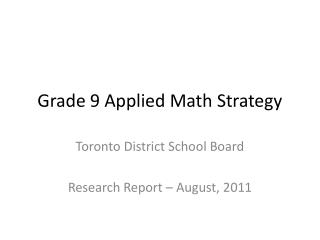Download Presentation# Grade 9 Applied Math Strategy - PowerPoint PPT PresentationDownload Presentation## Grade 9 Applied Math Strategy

- - - - - - - - - - - - - - - - - - - - - - - - - - - E N D - - - - - - - - - - - - - - - - - - - - - - - - - - -
##### Presentation Transcript

1. Grade 9 Applied Math Strategy Toronto District School Board Research Report – August, 2011

2. The Gap Closing students (GC) had a higher percentage change from PRE-test to POST-test (M=5.8%, SD=(16%) than Non-Gap Closing students (NGC) (M=-2.1%, SD=19%). This difference was significant, t(300) = 3.12, p < .05, two-tailed.

3. Analysis of Variance revealed a significant difference between the four Gap-Closing groups - No Intervention, Whole Class intervention, Small Group intervention, and Individual Intervention, F(3, 298) = 3.97. Further analysis showed that the group that received No intervention had a significantly lower percentage change than the group that had Individual intervention, t(70) = -2.82, p <.05, two-tailed; than the group that had Whole Class intervention, t(238) = -2.78, p<.05, two tailed; and than the group that had Small Group intervention, t(94) = -2.11, p<.05, two-tailed. No other significant differences were found. [this means that there is no statistical difference between any of the three groups that received gap-closing]

4. Gap-Closing Males (GC Males) and Gap-Closing Females (GC females) showed no significant differences in their percentage change from PRE-test to POST-test. t(248) = 0.47, p > .05

5. Student Survey: Math is Boring Results: Students who used Gap Closing Strategy

6. Student Survey: I like math. Results: Students who used Gap Closing Strategy

7. Student Survey: Students so well in math because they have natural ability. Results: Students who used Gap Closing Strategy

8. Student Survey: When I have trouble with a math question I create a diagram or make a model. Results: Students who used Gap Closing Strategy

9. Student Survey: When I struggle with a math problem, I give up. Results: Students who used Gap Closing Strategy

10. Student Survey: To learn mathematics, I try to remember every step in a procedure. Results: All students.

11. Teacher Survey Results

12. Teacher Survey Results